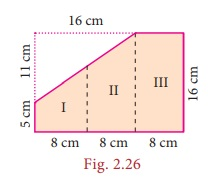Home | | Maths 8th Std | Perimeter and Area of Combined shapes - Example solved problems

# Perimeter and Area of Combined shapes - Example solved problems

8th Maths : Chapter 2 : Measurements : Perimeter and Area of Combined shapes: Numerical Example solved problems

Example 2.5

Find the perimeter and area of the given Fig.2.23. (π = 22/7)Solution:

Radius of a circular quadrant, r = 3.5 cm and side of a square, a = 3.5 cm.

The given figure is formed by the joining of 4 quadrants of a circle with each side of a square. The boundary of the given figure consists of 4 arcs and 4 radii.

(i) Perimeter of the given combined shape

= 4 × length of the arcs of the quadrant of a circle + 4 × radius= 22 + 14 = 36 cm (approximately)

(ii) Area of the given combined shape

= area of the square + 4 × area of the quadrants of the circleA = 12.25+38.5 = 50.75 cm2 (approximately)

Example 2.6

Nishanth has a key-chain which is in the form of an equilateral triangle and a semicircle attached to a square of side 5 cm as shown in the Fig. 2.24. Find its area. ( π = 3.14, √3 = 1.732)Solution:

Side of the square = 5 cm

Diameter of the semi-circle = 5 cm Radius = 2.5 cm

Side of the equilateral triangle = 5 cm

Area of the key-chain = area of the semi circle + area of the square + area of the equilateral triangle= 9.81+ 25 + 10.83

= 45.64 cm2 (approximately)

Example 2.7

A 3-fold invitation card is given with measures as in the Fig. 2.25. Find its area.Solution:

Figures I and II are trapeziums separately as well as combinedly.

The parallel sides of the combined trapezium (I and II) are 5 cm and 16 cm and its height, h = 8 + 8 = 16cm , length of the rectangle (III) = 16 cm and its breadth = 8 cm

Area of the combined invitation card = area of the combined trapezium + area of the rectangle

= 1/2 ×h×(a +b)+ l ×b= 1/2×16×(5 +16)+16×8

= 168 + 128 = 296 cm2

Aliter:

Area of the invitation card = area of the outer rectangle – area of the right angled triangle

= [l ×b] [ 1/2 ×b×h ]

= [ 24×16 ] [ 1/2 ×11×16 ]

= 38488 = 296 cm2Example 2.8

Seenu wants to buy a floor mat for his kitchen at home as given in Fig. 2.27. If the cost of the mat is 20 per square foot, what will be the cost of the entire mat?Solution:

The mat given in the figure can be split into two rectangles as follows:

Area of the entire mat = area of the I rectangle + area of the II rectangle

= l1 ×b1 + l2 ×b2

= 5 ×2 + 9 ×2 = 10 +18 =28 sq.feet

Cost per sq. foot = 20The total cost of the entire mat = 28 × 20 = 560

Try these

In the above example split the given mat into two trapeziums and verify your answer.

Solution:

Area of the mat = Area of I trapezium + Area of II trapezium

= [ 1/2 × h1 × (a1 + b1 )] + [ 1/2 × h2 × (a2 + b2 )] sq. units

= [ 1/2  × 2 × (7 + 5)] + 1/2 × 2 × (9 + 7) sq. feet

= 12 + 16 = 28 sq.feet

Cost per sq.feet =  ₹ 20

Cost for 28 sq. feet =  ₹20 × 28 = ₹560

Total cost for the entire mat = ₹560

Both the answers are the same.

Example 2.9

Find the area of the shaded region in the square of side 10 cm as given in the Fig. 2.29. (π = 22/7)Solution:

Mark the unshaded parts of the given figure as I, II, III and IV

Area of the I and III parts = Area of the square – Area of 2 semicircles= 10×10 22/7 ×5×5 = 100 – 78.57 = 21.43 cm2.

Similarly, the area of the II and IV parts = 21.43 cm2

Area of the unshaded parts (I, II, III and IV)

= 21.43 ×2 = 42.86 cm2 (approximately)

Area of the shaded part = area of the square – area of the unshaded parts

= 100 – 42.86 = 57.14 cm2 (approximately)

Do you Know:

1. The area of the unshaded regions in each of the squares of side a units are the same in all the cases given below.2. If the biggest circle is cut from a square of side ‘aunits, then the remaining area in the square is approximately 3/14 a2 sq.units. ( π = 22/7)

3. The area of the biggest circle cut out from the square of ‘a’ units = 11/14 a2 sq. units (approximately)

4. In the given figure if π = 22/7 , the area of the unshaded part of a square of side a units is approximately 3/7 a2 sq.units and that of the shaded part is approximately 4/7 a2 sq.units.Example 2.10

Find the area of an irregular polygon field whose measures are as given in the Fig. 2.30.Solution:

The given field has four triangles (I, III, IV and V) and a trapezium (II).∴ The total area of the field = 15 + 65 + 16 + 65 + 65 = 226 m2

Tags : Questions with Answers, Solution | Measurements | Chapter 2 | 8th Maths , 8th Maths : Chapter 2 : Measurements
Study Material, Lecturing Notes, Assignment, Reference, Wiki description explanation, brief detail
8th Maths : Chapter 2 : Measurements : Perimeter and Area of Combined shapes - Example solved problems | Questions with Answers, Solution | Measurements | Chapter 2 | 8th Maths